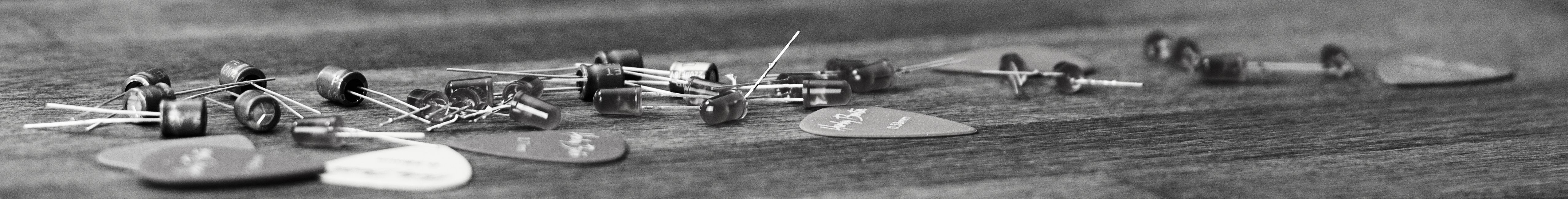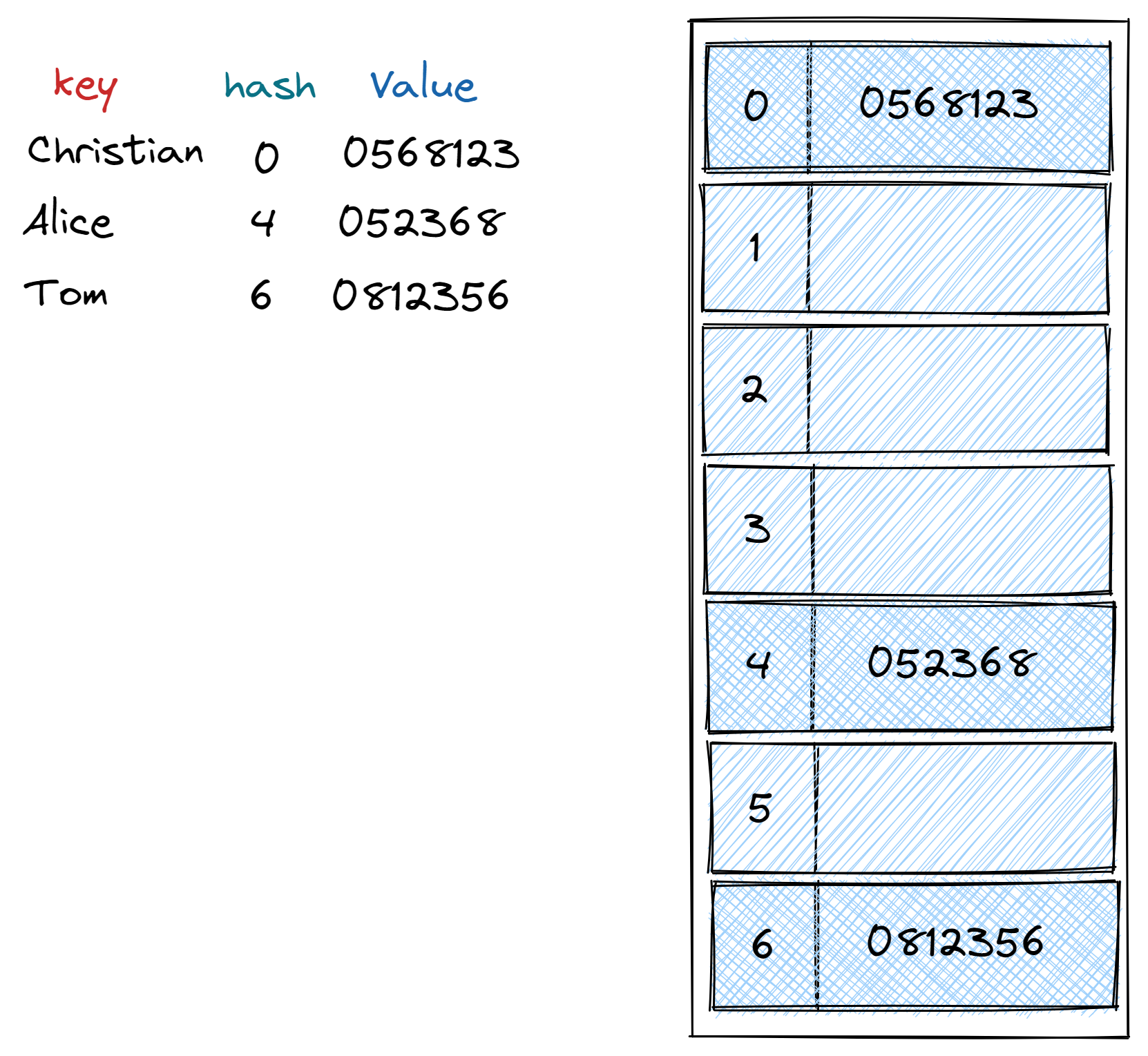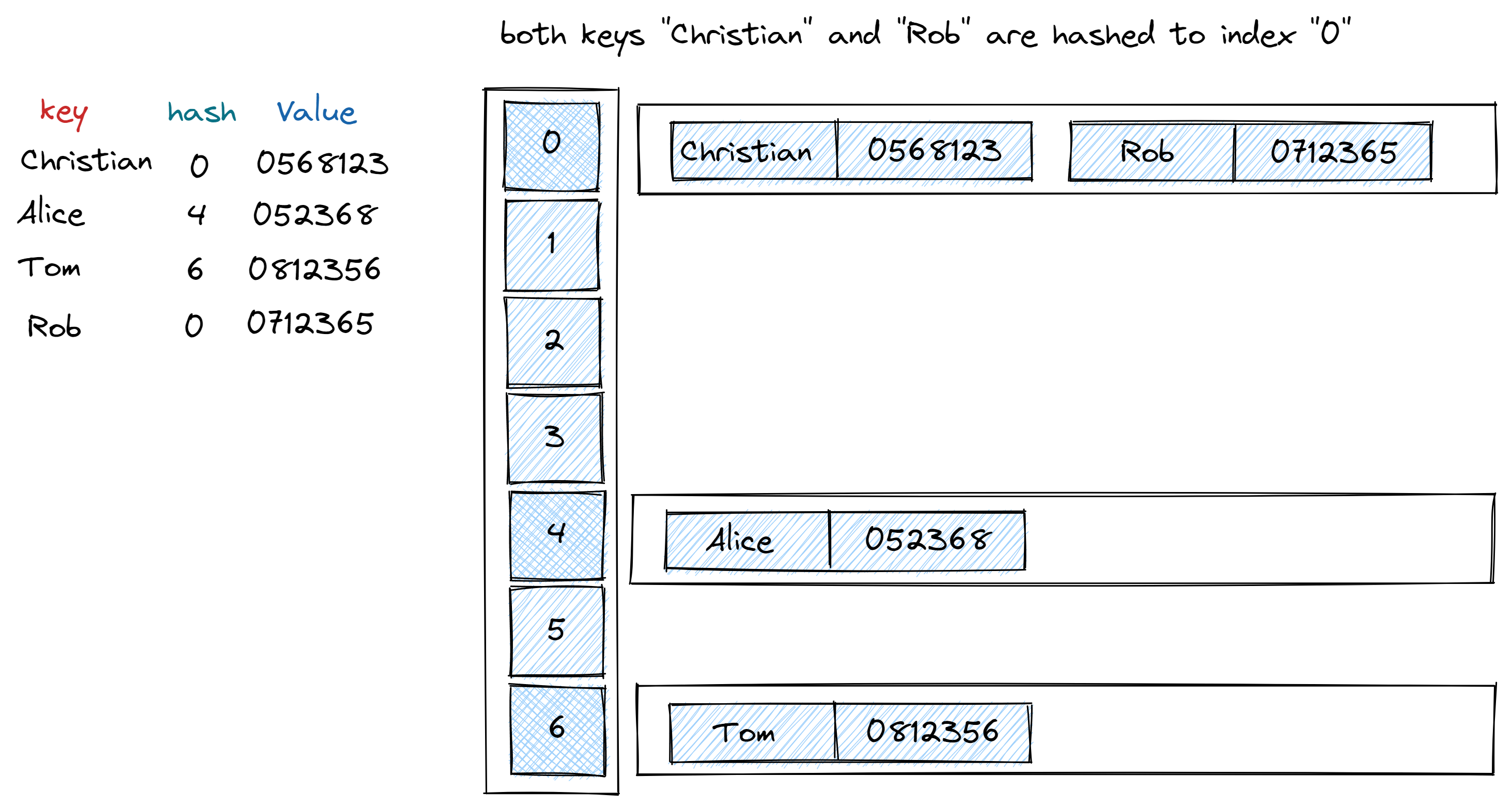savchenko.tech / software engineering blog by Maryna Savchenko## Hash table. Algorithms series

2022-08-01

Dictionary operations (INSERT, SEARCH and DELETE) are required by many applications. One very effective data structures for implementing dictionaries is hash table. The average time to search for an element in hash table is O(1).

Hash table ia unordered symbol table where key is interpreted as an array index and value associated with key ‘i’ is stored in array entry ‘i’.Two main parts in hash algorithm are hash function and collision resolution method.

### HASH FUNCTION

There are 3 primary requirements for implementing a good hash function:

• it should be consistent - equal keys must produce the same hash value.
• it should be efficient to complete.
• it should uniformly distribute the keys.

We can check consistency and distribution with a simple test:

``````    @Test
void shouldHashKey() {
HashTable<String, Integer> hashTable = new HashTable<>(7);
int christianCode = hashTable.hash("Christian");
assertEquals(0, christianCode);

int davidCode = hashTable.hash("David");
assertEquals(2, davidCode);

int aliceCode = hashTable.hash("Alice");
assertEquals(4, aliceCode);

int tomCode = hashTable.hash("Tom");
assertEquals(6, tomCode);

}
``````
``````    protected int hash(Key key) {
int h = key.hashCode();
h ^= (h >>> 20) ^ (h >>> 12) ^ (h >>> 7) ^ (h >>> 4);
return h & (tableSize - 1);
}
``````

### COLLISION RESOLUTION

Collision resolution handles the case when two or more keys to be inserted hash to the same index. Straightforward strategy for collision resolution is separate chaining. For each index in array there is a data structure like Linked List or a Tree Map and keys from this data structure are hashed to this index.Implementation of this kind of hash table is not too complicated. So in test cases we need to check that:

• inserted value is present
• deleted value is absent
``````    @Test
void insertedValueShouldBePresent() {
HashTable<String, Integer> hashTable = new HashTable<>(5);
hashTable.put("Alice", 123654);

assertEquals(123654, hashTable.get("Alice"));
}

@Test
void removedValueShouldBeAbsent() {
HashTable<String, Integer> hashTable = new HashTable<>(5);
hashTable.put("Alice", 123654);
hashTable.put("David", 456123);

hashTable.delete("Alice");
assertNull(hashTable.get("Alice"));
}
``````
``````    public void put(Key key, Value value) {
if (value == null) {
delete(key);
return;
}
if (keyValuePairsNumber >= 10 * tableSize) resize(2 * tableSize);

int i = hash(key);
if (!chains[i].containsKey(key)) keyValuePairsNumber++;
chains[i].put(key, value);
}

public Value get(Key key) {
int i = hash(key);
return chains[i].get(key);
}

public void delete(Key key) {
int i = hash(key);
if (chains[i].containsKey(key)) keyValuePairsNumber--;
chains[i].remove(key);

if (keyValuePairsNumber <= 2 * tableSize) resize(tableSize / 2);
}
``````

Complete implementation with test cases can be found on my GitHub.

Sources: